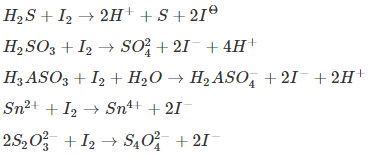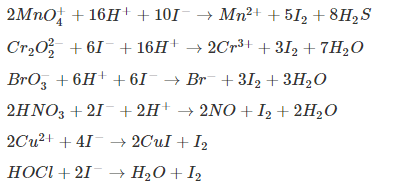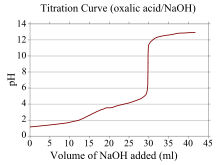Win up to 100% scholarship on Aakash BYJU'S JEE/NEET courses with ABNAT Win up to 100% scholarship on Aakash BYJU'S JEE/NEET courses with ABNAT

# Titration

## What Is Titration?

Titration, also known as titrimetry, is a chemical qualitative analysis technique that is used to calculate the concentration of a given analyte in a mixture. Titration is an important technique in the field of analytical chemistry and is also referred to as volumetric analysis.

### Titration Procedure

The process of titration involves the preparation of a titrant/titrator, which is a standard solution whose volume and concentration are predetermined. This titrant is then made to react with the analyte until some endpoint or equivalence point is reached; at that stage, the concentration of the analyte can be determined by measuring the amount of titrant consumed. Alternatively, titration is the concept of stoichiometry that is applied to find the unknown concentration of a solution.

As the first step of the procedure, a very precise amount of the analyte is added to a beaker or Erlenmeyer flask. A small amount of indicator (such as phenolphthalein) is placed underneath a calibrated burette or chemistry pipetting syringe, which consists of the titrant.

Small volumes of the titrant are added to the analyte and indicator. This will go on until the indicator changes colour in reaction to the titrant saturation threshold. At this point, it will represent that we have come to the endpoint of the titration. Basically, in this case, the amount of titrant balances the amount of analyte present during the reaction.

### Preparation Techniques

The titrant and analyte have to be in a liquid (solution) form. Solvents such as glacial acetic acid or ethanol are used to dissolve the solids. Concentrated analytes are also diluted to improve accuracy. In most of the non-acid–base titrations, a constant pH is required or should be maintained during the reaction. For this, a buffer solution is added to the titration chamber to maintain the pH.

A separate masking solution is sometimes added for certain conditions in the reaction chamber, and this eliminates the effect of the unwanted ion. Some redox reactions require heating the sample solution and titrating while the solution is still hot to increase the reaction rate.

## Chemical Analysis

The world of chemical analysis can be divided into two basic types.

• Qualitative Analysis: Where one finds the composition of a compound, i.e. to find what radicals are present in the salt.
• Quantitative Analysis: Where the interest is laid upon the concentration of an unknown solution.

There are some fundamental requirements that should be understood before one does the titration. The unknown concentration of a solution can be in the form of an acid, base or salt of an acid or base. For any titration process, the method is similar except for a few differences. The titration process can be classified into the following ways:

• Gravimetric Analysis
• Volumetric Analysis
• Combustion Analysis
• Others (Spectroscopy)

Titrations can also be used to furnish the purity of samples, calculation regarding PH, etc. Any calculations during the analysis can be done in two ways.

1. Using mole concepts (Required balanced chemical equations)
2. Using equivalent concepts (Balanced chemical equations are not required)

Therefore, a sound knowledge of the concepts of moles and equivalents is mandatory before studying titration. In this article, wherever required, the above-said concepts are included for convenience.

## Types of Titrations

Depending on the types of reactions involved, titrations can be classified as follows:

There can be cases where the titrate can have more than one component (For example, Na2CO3 + NaHCO3). Therefore, based on the number of components in the titrate, the titrations can be divided as follows.

• Single Titration
• Double Titration

## Acid-Base Titration (Acidimetoy or Alkalimetry)

Acid-base titrations mainly depend on the neutralization between an acid and a base when mixed in solution. More significantly, the strength of an acid is determined by using a standard solution of a base. This process is also called acidimetry.

Acids can be classified into strong or weak acids depending on the amount of dissociation to give H+ ions when dissolved in water. If an acid solution of known concentration is titrated against a strong base, the concentration of acid can be calculated, considering the fact that the neutralization reaction reaches completion. For the same fact, only a strong base is used for the titration process. So, in this case, the acid solution is the titrate, and the strong base is the titrant or the standard solution.

### The Procedure of Acid-Base Titration

• The required volume of the base is taken, whose concentration is known in a pipette and is poured into the titrating flask.
• The acid whose concentration is unknown is taken in the burette and is allowed to react with the base drop by drop.
• An indicator that is used for detecting the endpoint is also added to the titration flask.
• When the reaction reaches completion, the colour of the solution in the titration flask changes due to the presence of the indicator.
• The indicator used for this purpose can be phenolphthalein which forms pink colour in basic solution and is colourless in acid and neutral solution.

Therefore, the endpoint is detected when the pink-coloured solution turns colourless.

### How to Choose an Indicator for an Acid-Base Titration?

When the acid is very weak, the detection or obtaining the endpoint is difficult. For this purpose, the salt of the weak acid is titrated against a strong acid because the conjugate base of a weak acid is a strong base.

For example, CH3COOH is a weak acid. But CH3COONa is a strong base. Examples of strong acids are HNO3, HCl, H2SO4, and HClO3.

Bases are of two types: strong and weak. The same process is done in the case of acid titration except that the unknown solution (titrate) is the base and titrant is a strong acid. Indicators used in this case can be methyl red or methyl orange, which is orange in acidic solutions and yellow in basic and neutral solutions.

As in the case of acids, in order to obtain 100% completion of the reaction, a weak base is first converted into its salt and titrated against a strong base.

If a salt of a weak acid and weak base like ammonium carbonate’s concentration has to be found out, it can be titrated against a strong acid or a strong base depending on the salt.

The analysis part in which the calculation of unknown concentration is illustrated in the following example.

A 2.0 g sample of an unknown monobasic acid is dissolved in 100 ml water, and 20 ml portion of this solution required 15 ml of 0.12 m NaOH solution to reach the endpoint. If the molecular mass of acid is 122g/mol, determine the purity % of the acid.

Solution:

For 20 ml acid solution, 15 ml of 0.12 m NaOH is required

Therefore, number of equivalents of base = 12 × 15 = 1.8 × 10-3 equivalents

Therefore, in 20 ml of the acid solution, 1.8 0 × 10-3 equivalents of acids are present

Therefore, if 20ml → to 1.8 × 10-3 equivalents for 100 ml → X

Therefore, x = 9 × 10–3 is equivalent since it is a monobasic acid, and the molar mass acid equation mass is the same.

In 2g of sample:

Mass of acid = 9 × 10-3 × 122 = 1.098 g

% purity = 1098/2 × 100 = 54.9%.

## Double Titration

In this type of titration, the titrate (unknown concentration) solution contains more than one component.

Examples can be a mixture of NaOH and Na2CO3 or Na2CO3 and NaHCO3

To find the composition of the mixture or to check the purity of a sample, titration of the mixture is done against a strong acid. But in this case, instead of one indicator, two indicators are used because there are going to be two endpoints during the titration. Commonly used indicators are phenolphthalein and methyl orange. Before understanding how to calculate, getting familiar with the reactions involved is important. In the case of a mixture of two bases like NaOH + Na2CO3 or Na2CO3 + NaHCO3, first, the stronger bases will react with the acid to reach the 1st endpoint and then only the weaker base.

## Redox Titration

These types of titrations are almost similar to the volumetric acid. Base titrations except that here, the reactions involved are Redox reactions. Here, the interest is laid upon finding the unknown concentration of a reducing or an oxidizing agent. The oxidizing or reducing agents are titrated against strong reducing or oxidizing agents, respectively. In most of the redox titrations, one of the reactants itself will act as an indicator (self indicators), changing their colour with reference to their oxidizing states.

Equivalents weights of oxidizing and reducing agents

Balancing a redox reaction is a tedious job, so using the concept of equivalents is very much preferred in redox titrations.

### Oxidation Titration Using KMnO4

KMnO4 is a strong oxidizing agent, which almost oxidizes every other common reducing agent. It is purple in colour and changes to colourless when Mn2+ or

$$\begin{array}{l}MnO_{4}^{2-}\end{array}$$
is formed and black when it forms MnO2.

Redox titration, also known as an oxidation-reduction reaction, is a chemical reaction that mostly occurs with a transfer of electrons in the reacting ions of aqueous solutions. The titrations are further named after the reagent that is used in them, and they are as follows:

• Permanganate Titrations
• Dichromate Titrations
• Iodimetric and Iodometric Titrations

## Iodometric and Iodimetric Titration

This titration involves iodine, and iodide solutions are used as titrants. The use of iodine or iodide can be done in two ways:

### Iodimetry Titration (Direct Method)

In this type of titration, iodine solution (I2) is used for titrations of reducing agents. The following are common reactions involving iodine.Common reactions involved in iodimetry titration

In all the reactions, I2 acts as a weak oxidizing agent.

### Iodometry Titration (Indirect Method)

Here, Iacts as a reducing agent, which is oxidized to I2. The amount of I2 is titrated against standard hypo (thiosulphate) solution, which in turn is used for estimating the unknown concentration of the reaction oxidizing agent. The following are some common reactions involved:Common reactions involved in iodometry titration

## Gravimetric Analysis Titration

This is done for elements that can form insoluble salts in an aqueous solution. It involves the separation of ions from the compound in the form of precipitates. The following are the steps involved in the process.

1. A sample of a certain amount is taken.
2. The required component in the sample is converted into a precipitate.
3. This precipitate is purified and tested, and the required result (concentration or purity of the sample) is obtained.

### Endpoints or Equivalence Point

This is the stage where the reaction attains completion. Endpoints or equivalence points can also be defined as a point in the reactions where the number of equivalents of titrant and titrate becomes equal.

To detect the endpoint, most of the SP ime and “Indicator” is used. The indicator used depends on the type of reaction.

For example, phenolphthalein or methyl orange can be used for titrations involving acids and bases. The indicators indicate the endpoint by changing their colours at the endpoint; sometimes, one of the reactants itself can act as an indicator.

## Volumetric Analysis Titration

This involves two reactants of known volumes; the concentration of one of the solutions is known, and the other is unknown. The reaction involved in the titration process should satisfy the following criteria.

1. The skeletal equation of the reaction should be known.
2. The reaction should not be too slow or fast so that the calculations are free of error.
3. The endpoint (completion) of the reaction should be easily detectable. For this purpose, indicators are used, which are explained later.
4. There should not be any side reactions. If there is something that makes up the side reactions, that particular component should be removed before the titration process.

Basic Requirements for Titration:

For any titration process, there are some basic definitions that are important.

1. Titre or titrate or analytic: This is the solution whose concentration is unknown.
1. Titrant: This is the solution whose concentration is known.

Standard Solution: The titrant is actually taken from the standard solution whose concentration is known. Depending on how they are prepared, they can be of two types:

Primary standard

This is prepared by dissolving a measured amount of solute in a measured volume of water directly. For example, ferrous ammonium sulphate.

Secondary standard

This is prepared by titrating a solution against a primary standard solution of KOH, NaOH, or any other which can’t be stored for a longer duration and comes under the secondary standard.

A standard solution should satisfy the following criteria:

1. The purity of the sample should be ideally 100%
2. Stable at room temperature
3. Concentration should not change over a period of time
4. It should have a high molecular mass. This gives error-free calculations.

### Gas-phase titration

These are titrations done in the gas phase, specifically as methods for determining reactive species by reaction with an excess of some other gas acting as the titrant.

### Complexometric titration

Complexometric titrations mainly depend on the formation of a complex between the analyte and the titrant.

### Zeta potential titration

Zeta potential titrations are those where the completion of the reaction is monitored by the zeta potential rather than by an indicator in order to characterise heterogeneous systems, such as colloids.

### Assay

An assay is a type of biological titration that is mainly used for the determination of the concentration of a virus or bacterium.

## Titration Curve

A titration curve is where we plot the pH of the analyte solution versus the volume of the titrant added as the titration progresses. In a titration curve, the x-coordinate of which represents the volume of titrant added since the beginning of the titration. The y-coordinate represents the concentration of the analyte at the corresponding stage of the titration. In an acid-base titration, the titration curve mostly represents the strength of the corresponding acid and base.